### Lines and Angles - Test Papers

CBSE Test Paper 01

CH-6 Lines and Angles

1. In the adjoining figure, AB  CD and AB  EF. If EA  BA and BEF = 55°, then the values of x, y and z :-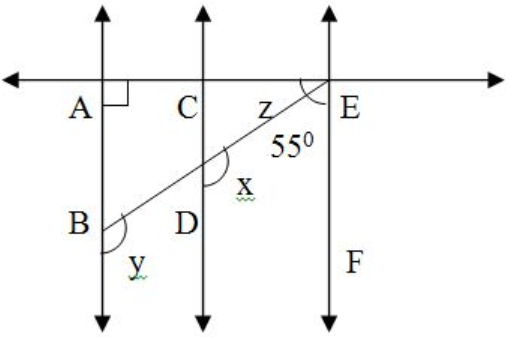1. 125°, 125°, 35°
2. 60°, 60°, 60°
3. 120°, 130°, 25°
4. 35°, 125°, 120°
2. If one angle of a triangle is equal to the sum of the other two angles, then the triangle is :
1. an isosceles triangle
2. an equilateral triangle
3. a right triangle
4. an obtuse angled triangle
3. In the adjoining figure, if QP  RT, then x is equal to –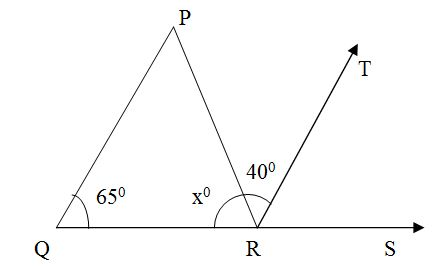1. 55°
2. 75°
3. 65°
4. 70°
4. The number of lines that can pass through a given point is:
1. only one
2. two
3. one
4. Infinity
5. In figure, PQ  RS,  QPR = 70°,  ROT = 20° find the value of x.1. 20°
2. 70°
3. 50°
4. 110°
6. Fill in the blanks:

An equation of the type ________ represents a straight line passing through the origin.

7. Fill in the blanks:

The common between the three angles of a triangle and a linear pair is ________.

8. In Fig., find the value of x for which the lines l and m are parallel.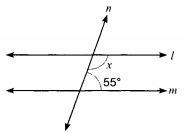9. Find the measure of the complementary angle of 60o.

10. In the given figure ABC is right angled at A. AD is drawn perpendicular to BC. Prove that BAD=ACB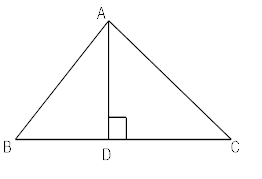11. Prove that if one angle of a triangle is equal to the sum of other two angles, then the triangle is right angled.

12. The exterior angle of a triangle is 110° and one of the interior opposite angle is 35°. Find the other two angles of the triangle.

13. AB, CD and EF are three concurrent lines passing through the point O such that OF bisects BOD. If  BOF = 35o. Find BOC and AOD.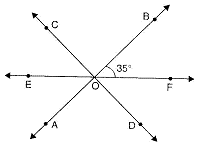14. In Fig., if ABCD,  APQ=50 and PRD=127, find x and y.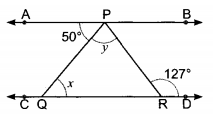15. In ABC in given figure, the sides AB and AC of  ABC are produced to points E and D respectively. If bisectors BO and CO of CBE and BCD respectively meet at point O, then prove that BOC = 90° - 12  A .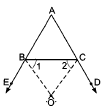CBSE Test Paper 01
CH-6 Lines and Angles

Solution

1. (a) 125°, 125°, 35°
Explanation: x + 55 = 180° (Sum of supplementary angles or co-interior angles)
x = 125°
x = y = 125° (Corresponding angles)
z + EAB = y (Exterior angle property)
z = 125° - 90° = 35°
2. (c) a right triangle
Explanation: The sum of the angles of triangle  is 180 degrees.
let the angles of triangle be a ,  b, c
we have given that one angle of a triangle is equal to the sum of the other two angles
so we have
c=a + b
a + b + c = 180
Substitute c for a +b
c + c = 180
2c = 180
c = 90
therefore the triangle is a right triangle.
3. (b) 75°
Explanation:
QPR = PRT = 40° (Alternate interior angles)
In QPR
PQR + QPR + PRQ = 180° (Angle sum property)
65° + 40° + x° = 180°
x° = 180° - 40° - 65°
x° = 75
4. (d) Infinity
Explanation: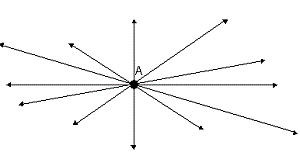As seen from the above image, any number of lines can be drawn through a given point.
Hence the answer may be given as "Infinity".

5. (c) 50°
Explanation:

PQ  RS
QPR = SRO = 70° (Corresponding, Angle)
NOW IN RTO
x + 20° = 70° (exterior angle)
x = 70° - 20°
x = 50°

6. y = mx

7. 180o

8. Two lines are parallel when angles on the same side of the transversal are supplementary i.e.,
x+55=180x=18055 x=125

9. The measure of the complementary angle x = (90o – ro)
Where ro = given measurement
∴ x = (90o – 60o) = 30o

from ABD
BAD = 90° - ABD ..(i)
But A + B + C = 180° ABC
B + C = 900  A = 900
C = 90° - B ...(ii)
From (i) and (ii)

11. Given in ΔABC B = A + C

Proof: A+B+C=180o….. (1) [Sum of three angles of a ΔABC is 180°]
A+C=B….. (2)
From (1) and (2)
B+B=180o
2B = 180o
B = 90o

Hence, the triangle is a right angled triangle.

12. Since the exterior angle of a triangle is equal to the sum of interior opposite angles.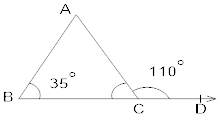ACD = A + B
110 = A + 35o
A = 110o35o
A = 75o
Now, A+B+C=180(Angle sum property)
C = 180(A + B)
C = 180(75o+35o)
C = 70o

13.OF bisects BOD . . . [Given]
BOF = DOF = 35o
COE = DOF = 35o
EOF = 180o . . . . [A straight angle = 180o]
EOC + BOC + BOF = 180o
35o + BOC + 35o = 180o
BOC = 180o – 70o = 110o
AOD = BOC . . . [Vertically opposite angles]
= 110o

14. As ABCD and PQ is a transversal.
APQ=PQR (Alternate interior angles)
50=x ...........(1)
Also, APR=PRD (Alternate interior angles) .
APQ+QPR=127°
x+y=127
50+y=127. [ From (1) ]
or ​​​, y = 127° - 50° = 77°
Hence, x = 50° & y = 77°

15.As ABC and CBE form a linear pair
ABC + CBE = 180°..........(1)
Given, BO is the bisector of CBE. Hence,
CBE = 2OBC.
CBE = 21...............(2)

Therefore, ABC+ 21 =180° [ from (1) & (2) ]
21 = 180° - ABC
1 = 90° - 12ABC........(3)
Again, ACB and BCD form a linear pair
ACB + BCD =180°.........(4)
Given, CO is the bisector of BCD.  Hence,
BCD = 22............(5)
So, ACB + 22 = 180° [ from (4) & (5) ]
2 2 = 180°- ACB
2 = 90° - 12ACB ...(6)

Now in OBC, we have
1+ 2 + BOC = 180° (Angle sum property of triangle) ...(7)

From (3), (6) and (7), we have
90°- 12ABC + 90°- 12ACB + BOC = 180° .
BOC = 12(ABC + ACB )...........(8)
Now, in ABC, we have
BAC + ABC + ACB = 180°
or, ABC + ACB = 180° - BAC............(9)

From (8) and (9), we have:-
BOC = 12(180° - BAC)
Hence, BOC = 90° - 12 A Proved.